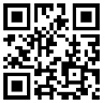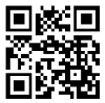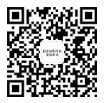•截图，长按识别二维码关注^_^
•截图，长按识别二维码关注^_^
•截图，长按识别二维码关注^_^
•截图，长按识别二维码关注^_^
•截图，长按识别二维码关注^_^
•截图，长按识别二维码关注^_^
•截图，长按识别二维码关注^_^
•截图，长按识别二维码关注^_^
•截图，长按识别二维码关注^_^
•截图，长按识别二维码关注^_^
•截图，长按识别二维码关注^_^
•截图，长按识别二维码关注^_^
•截图，长按识别二维码关注^_^
•截图，长按识别二维码关注^_^
•截图，长按识别二维码关注^_^
•截图，长按识别二维码关注^_^
•截图，长按识别二维码关注^_^
•截图，长按识别二维码关注^_^
•截图，长按识别二维码关注^_^
•截图，长按识别二维码关注^_^
•截图，长按识别二维码关注^_^
•截图，长按识别二维码关注^_^
•截图，长按识别二维码关注^_^
•截图，长按识别二维码关注^_^